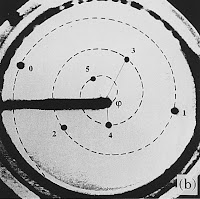## 24 September 2016

### Golden angle in plants: a mathematical illusion

According to Golden Number Mysticism,

φ = (√5-1)/2 = 0.61803....

is omnipresent in the universe. On planet Earth in particular, it is supposedly present in animals (for Nautilus shells, see here) and in plants. Leaves, seeds etc. are said to occur in patterns governed by an angle which is φ times a complete turn. Because this yields an angle greater than half a turn, we had as well turn 1-φ times in the other sense, and define the golden angle as

(1-φ)2π = 0.38196... x 360° = 137.50776...°

These botanical claims too are false. It is true that Fibonacci numbers occur, not as universally or unambiguously as claimed, but with a frequency that calls for an explanation. (We'll consider Fibonacci patterns next time.) The golden ratio, on the other hand, does not occur. In theoretical models of plants, the golden angle "occurs" as the mathematical limit of certain angles associated with Fibonacci numbers. These angles are themselves unrealistic mathematical idealizations, and so the golden angle is two huge steps away from observable reality. Wishful thinking does the rest.

1. There is no such thing as a constant divergence angle. Most botanical models (= idealizations, simplifications) rely on the assumption that successive elements (leaves, seeds etc.) are added around a point or axis of symmetry in such a way that the "divergence angle" between two new elements is constant. This cannot be real. The divergence angle reflects the Fibonacci pattern of the plant (which is real), but a plant switches from one Fibonacci pattern to the next while growing. Thus a sunflower head can have a middle zone (21,13) and an outer zone (21,34)  (photo here, p.32), and an asparagus stem can change from (2,3) to (5,8) (photo here, p.271). Clearly, the "divergence angle" has to be different for the two patterns, and certainly while the transition takes place. [See update on top of the page.]

2. "Real" divergence angles are ill defined and cannot be measured with any accuracy. To define an angle, three points are required: the apex and a point on each leg. In plants, none of these points is sufficiently well defined to yield a useful "measurement". We consider two illustrations, in which the authors have themselves drawn the legs of the supposed divergence angles.

2a. The first example (here, p.52) has a caption saying
Illustration of the divergence angle D=2πd.
We had Geogebra compute the two angles indicated by the author as being 2πd, and they differ by more than 10° (one value green, the other blue). Leaving the apex—ill-defined as it is—unchanged, we moved the three other points to the centers of quadrangles that we fitted as good as possible on top of the organic entities, which are only approximately rectilinear quadrangles. Two new values are obtained (in red). Only one of the four is close to the golden angle, but this could be easily changed in either direction by some extra shuffling and/or relocating the apex.

2b. The second example (here, p.9) has the following caption:
Pinus pinea: phyllotaxis 8/5, divergence φ-2; the primordia are numbered according to the order of their births, in the center of the figure (from Church, 1904a).
The legs of the angle were added by the author on top of the original figure in Church (here, p.255) Same remarks as above: the angle is very coarsely defined, and its actual value (courtesy of Geogebra) is far removed from the golden value that is explicitly claimed by the author.

3. "Mathematical" divergence angles are so defined in terms of the Fibonacci pattern, that they converge automatically to the golden angle.

Authors may have an own formula defining their preferred divergence angle (after all, disproving by measurement is all but impossible), but here is a typical example (here, p.11).

The first column gives a sequence of number pairs, each one consisting of two successive Fibonacci numbers, say (fn,fn+1). In the second column, the corresponding "divergence angle" is given, which is nothing else but (fn/fn+2) times a complete circle. It is well known that the ratio of two successive Fibonacci numbers converges to φ, hence (fn/fn+2) converges to φ2=1-φ. This means that the angles in the second column inevitably converge to the golden angle.

4. There is a desire to spot the golden value. Here (p.3), in a discussion of the Douady-Couder experiment (to be considered next time) by the Dutch Biology Institute, we read
At higher speeds something remarkable happened. When a drop fell in the oil, it experienced repulsive forces from a number of preceding drops, creating a spiral-like pattern. The angle between the lines along which two successive drops flowed away, was always the same: 137.5°!
and the golden angle is proudly displayed in their diagram (right part of the image)

Alas, this is wishful thinking. Here is what Douady-Couder actually write (here, p.259, cases (b) and (c)):
(b) Below the first symmetry breaking bifurcation the successive drops move away from each other with a divergence angle φ=150° (shown between drop three and four). They define an anti-clockwise generative spiral (dashed line). The parastichy numbers correspond to (1,2). (c) For smaller advection higher order Fibonacci modes are obtained. Here φ≈139° and the parastichy numbers are (5,8).
They give their angles, 150° and 139°, without decimals, indicating low precision. Sure enough, if you measure them geometrically, you find a difference close to 1°, but in any case a value far away from the golden value. (Left as defined by Douady-Couder, right measured by Geogebra.)Summary.

1. Plants don't have a fixed divergence angle, let alone a golden one.

2. "Measurements" of divergence angles cannot yield anything more precise than a wide range of values bound to include the golden value (which reflects the mathematical limit of the Fibonacci pattern).

3. In numerical clouds, golden angles are spotted by golden angle spotters.

*